# 6.4 Special products  (Page 2/5)

 Page 2 / 5

Multiply: ${\left(4{u}^{3}+1\right)}^{2}.$

## Solution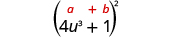Use the pattern.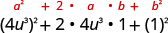Simplify.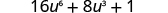Multiply: ${\left(2{x}^{2}+1\right)}^{2}.$

$4{x}^{4}+4{x}^{2}+1$

Multiply: ${\left(3{y}^{3}+2\right)}^{2}.$

$9{y}^{6}+12{y}^{3}+4$

## Multiply conjugates using the product of conjugates pattern

We just saw a pattern for squaring binomials that we can use to make multiplying some binomials easier. Similarly, there is a pattern for another product of binomials. But before we get to it, we need to introduce some vocabulary.

What do you notice about these pairs of binomials?

$\begin{array}{ccccccc}\hfill \left(x-9\right)\left(x+9\right)\hfill & & & \hfill \phantom{\rule{4em}{0ex}}\left(y-8\right)\left(y+8\right)\hfill & & & \hfill \phantom{\rule{4em}{0ex}}\left(2x-5\right)\left(2x+5\right)\hfill \end{array}$

Look at the first term of each binomial    in each pair.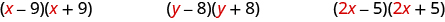Notice the first terms are the same in each pair.

Look at the last terms of each binomial in each pair.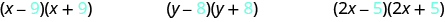Notice the last terms are the same in each pair.

Notice how each pair has one sum and one difference.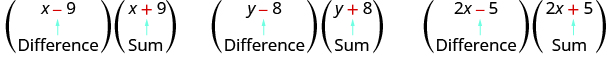A pair of binomials that each have the same first term and the same last term, but one is a sum and one is a difference has a special name. It is called a conjugate pair and is of the form $\left(a-b\right),\left(a+b\right)$ .

## Conjugate pair

A conjugate pair    is two binomials of the form

$\left(a-b\right),\left(a+b\right).$

The pair of binomials each have the same first term and the same last term, but one binomial is a sum and the other is a difference.

There is a nice pattern for finding the product of conjugates. You could, of course, simply FOIL to get the product, but using the pattern makes your work easier.

Let’s look for the pattern by using FOIL to multiply some conjugate pairs.

$\begin{array}{ccccccc}\hfill \left(x-9\right)\left(x+9\right)\hfill & & & \hfill \phantom{\rule{4em}{0ex}}\left(y-8\right)\left(y+8\right)\hfill & & & \hfill \phantom{\rule{4em}{0ex}}\left(2x-5\right)\left(2x+5\right)\hfill \\ \hfill {x}^{2}+9x-9x-81\hfill & & & \hfill \phantom{\rule{4em}{0ex}}{y}^{2}+8y-8y-64\hfill & & & \hfill \phantom{\rule{4em}{0ex}}4{x}^{2}+10x-10x-25\hfill \\ \hfill {x}^{2}-81\hfill & & & \hfill \phantom{\rule{4em}{0ex}}{y}^{2}-64\hfill & & & \hfill \phantom{\rule{4em}{0ex}}4{x}^{2}-25\hfill \end{array}$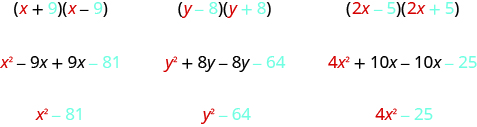Each first term is the product of the first terms of the binomials, and since they are identical it is the square of the first term.

$\begin{array}{}\\ \hfill \left(a+b\right)\left(a-b\right)={a}^{2}-\text{____}\hfill \\ \hfill \text{To get the}\phantom{\rule{0.2em}{0ex}}\mathbf{\text{first term, square the first term}}.\hfill \end{array}$

The last term came from multiplying the last terms, the square of the last term.

$\begin{array}{}\\ \hfill \left(a+b\right)\left(a-b\right)={a}^{2}-{b}^{2}\hfill \\ \hfill \text{To get the}\phantom{\rule{0.2em}{0ex}}\mathbf{\text{last term, square the last term}}.\hfill \end{array}$

What do you observe about the products?

The product of the two binomials is also a binomial! Most of the products resulting from FOIL have been trinomials.

Why is there no middle term? Notice the two middle terms you get from FOIL combine to 0 in every case, the result of one addition and one subtraction.

The product of conjugates is always of the form ${a}^{2}-{b}^{2}$ . This is called a difference of squares.

## Product of conjugates pattern

If $a\phantom{\rule{0.2em}{0ex}}\text{and}\phantom{\rule{0.2em}{0ex}}b$ are real numbers,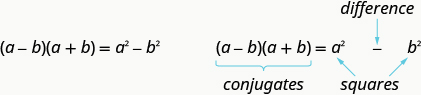The product is called a difference of squares.

To multiply conjugates, square the first term, square the last term, and write the product as a difference of squares.

Let’s test this pattern with a numerical example.

$\begin{array}{cccc}& & & \phantom{\rule{4em}{0ex}}\left(10-2\right)\left(10+2\right)\hfill \\ \text{It is the product of conjugates, so the result will be the}\hfill & & & \\ \text{difference of two squares.}\hfill & & & \phantom{\rule{4em}{0ex}}\text{____}-\text{____}\hfill \\ \text{Square the first term.}\hfill & & & \phantom{\rule{4em}{0ex}}{10}^{2}-\text{____}\hfill \\ \text{Square the last term.}\hfill & & & \phantom{\rule{4em}{0ex}}{10}^{2}-{2}^{2}\hfill \\ \text{Simplify.}\hfill & & & \phantom{\rule{4em}{0ex}}100-4\hfill \\ \text{Simplify.}\hfill & & & \phantom{\rule{4em}{0ex}}96\hfill \\ \text{What do you get using the Order of Operations?}\hfill & & & \\ & & & \phantom{\rule{4em}{0ex}}\left(10-2\right)\left(10+2\right)\hfill \\ & & & \phantom{\rule{4em}{0ex}}\left(8\right)\left(12\right)\hfill \\ & & & \phantom{\rule{4em}{0ex}}96\hfill \end{array}$

Notice, the result is the same!

Multiply: $\left(x-8\right)\left(x+8\right).$

## Solution

First, recognize this as a product of conjugates. The binomials have the same first terms, and the same last terms, and one binomial is a sum and the other is a difference.

 It fits the pattern.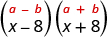Square the first term, x .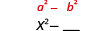Square the last term, 8.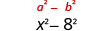The product is a difference of squares.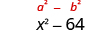p-2/3=5/6 how do I solve it with explanation pls
P=3/2
Vanarith
1/2p2-2/3p=5p/6
James
is y=7/5 a solution of 5y+3=10y-4
yes
James
Lucinda has a pocketful of dimes and quarters with a value of $6.20. The number of dimes is 18 more than 3 times the number of quarters. How many dimes and how many quarters does Lucinda have? Rhonda Reply Find an equation for the line that passes through the point P ( 0 , − 4 ) and has a slope 8/9 . Gabriel Reply is that a negative 4 or positive 4? Felix y = mx + b Felix if negative -4, then -4=8/9(0) + b Felix -4=b Felix if positive 4, then 4=b Felix then plug in y=8/9x - 4 or y=8/9x+4 Felix Macario is making 12 pounds of nut mixture with macadamia nuts and almonds. macadamia nuts cost$9 per pound and almonds cost $5.25 per pound. how many pounds of macadamia nuts and how many pounds of almonds should macario use for the mixture to cost$6.50 per pound to make?
Nga and Lauren bought a chest at a flea market for $50. They re-finished it and then added a 350 % mark - up Makaila Reply the sum of two Numbers is 19 and their difference is 15 Abdulai Reply 2, 17 Jose interesting saw Felecia left her home to visit her daughter, driving 45mph. Her husband waited for the dog sitter to arrive and left home 20 minutes, or 13 hour later. He drove 55mph to catch up to Felecia. How long before he reaches her? Rafi Reply integer greater than 2 and less than 12 Emily Reply 2 < x < 12 Felix I'm guessing you are doing inequalities... Felix Actually, translating words into algebraic expressions / equations... Felix hi Darianna hello Mister Eric here Eric He charges$125 per job. His monthly expenses are $1,600. How many jobs must he work in order to make a profit of at least$2,400?
at least 20
Ayla
what are the steps?
Alicia
6.4 jobs
Grahame
32
Grahame
1600+2400= total amount with expenses. 4000/125= number of jobs needed to make that min profit of 2400. answer is 32
Orlando
He must work 32 jobs to make a profit
POP
what is algebra
repeated addition and subtraction of the order of operations. i love algebra I'm obsessed.
Shemiah
hi
Krekar
Eric here. I'm a parent. 53 years old. I have never taken algebra. I want to learn.
Eric
One-fourth of the candies in a bag of M&M’s are red. If there are 23 red candies, how many candies are in the bag?
they are 92 candies in the bag
POP
rectangular field solutions
What is this?
Donna
t
muqtaar
the proudact of 3x^3-5×^2+3 and 2x^2+5x-4 in z7[x]/ is
?
Choli
a rock is thrown directly upward with an initial velocity of 96feet per second from a cliff 190 feet above a beach. The hight of tha rock above the beach after t second is given by the equation h=_16t^2+96t+190
Usman
Stella bought a dinette set on sale for $725. The original price was$1,299. To the nearest tenth of a percent, what was the rate of discount?
44.19%
Scott
40.22%
Terence
44.2%
Orlando
I don't know
Donna
if you want the discounted price subtract $725 from$1299. then divide the answer by $1299. you get 0.4419... but as percent you get 44.19... but to the nearest tenth... round .19 to .2 and you get 44.2% Orlando you could also just divide$725/\$1299 and then subtract it from 1. then you get the same answer.
OrlandoBy OpenStaxBy OpenStaxBy Rachel WoolardBy Jams KaloBy OpenStaxBy Rohini AjayBy OpenStaxBy OpenStaxBy OpenStaxBy Brooke Delaney